# 1-D model for precursor runs

The initial profiles of the horizontal wind components in PALM can be prescribed by the user by piecewise linear gradients or by directly using observational data. Alternatively, a 1-D model can be employed to calculate stationary boundary-layer wind profiles. This is particularly useful in neutral stratification, where inertial oscillations can persist for several days in case that non-balanced profiles are used for initialization. By employing the embedded computationally inexpensive 1-D model with a Reynolds-average based turbulence parametrization, these oscillations can be significantly damped. A stationary state of the wind profiles can thus be provided much faster in the 3-D model. The arrays of the 3-D variables are then initialized with the (stationary) solution of the 1-D model. These variables are ui where i ∈ {1, 2}, e, Kh, Km and, with MOST applied between the surface and the first vertical grid level, also L, u as well as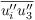(where i ∈ {1, 2}).

The 1-D model assumes the profiles of θ and qv, as prescribed by the user, to be constant in time. The model solves the prognostic equations for ui and e: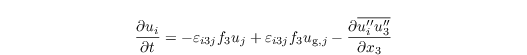and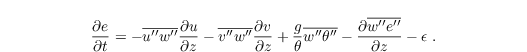The dissipation rate is parametrized by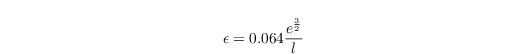after Detering and Etling (1985). The mixing length is calculated after Blackadar (1997) as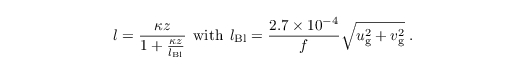The turbulent fluxes are calculated using a 1st-order closure: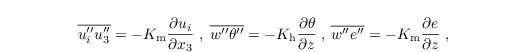where Km and Kh are calculated aswith the similarity functions Φh and Φm (see Eqs. in Sect boundary conditions), using the gradient Richardson number:Note that the distinction of cases in the Eq. above is done with the value of Ri from the previous time step.

Moreover, a Rayleigh damping can be switched on to speed up the damping of inertial oscillations. The 1-D model is discretized in space using finite differences. Discretization in time is achieved using the 3rd-order Runge--Kutta time-stepping scheme (Williamson, 1980). Dirichlet boundary conditions are used at the top and bottom boundaries of the model, except for e, for which Neumann conditions are set at the surface (see also Sect. boundary conditions).

• Detering HW, Etling D. 1985. Application of the E-ε turbulence model to the atmospheric boundary layer. Bound.-Lay. Meteorol. 33: 113–133.
• Blackadar AK. 1997. Turbulence and Diffusion in the Atmosphere. Springer. Berlin. Heidelberg. NewYork?. 185 pp.
• Williamson JH. 1980. Low-storage Runge–Kutta schemes. J. Comput. Phys. 35: 48–56.